0%

Waymo 行为预测的paper：《VectorNet: Encoding HD Maps and Agent Dynamics From Vectorized Representation》

## Overview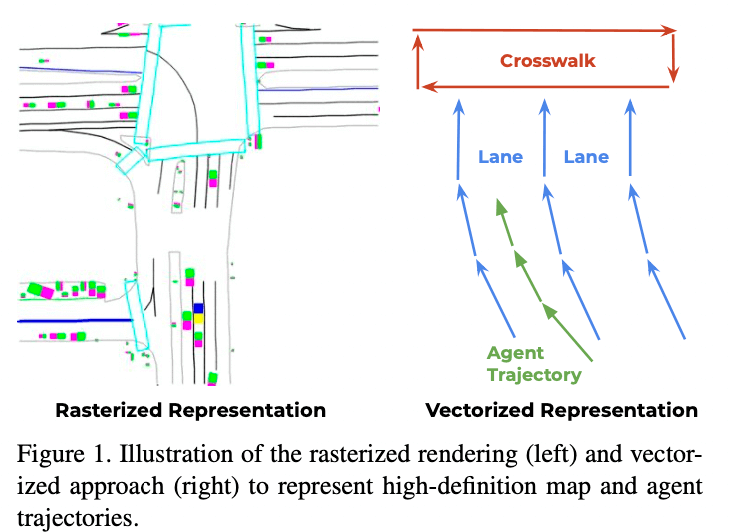vector-presentation-vs-rasterized-prepresentation

VectorNet在Argoverse和 in-house 的数据集上都取得了最好的效果，并且相比语义地图的方法节省70%的模型参数，节约了计算开销。

## 网络介绍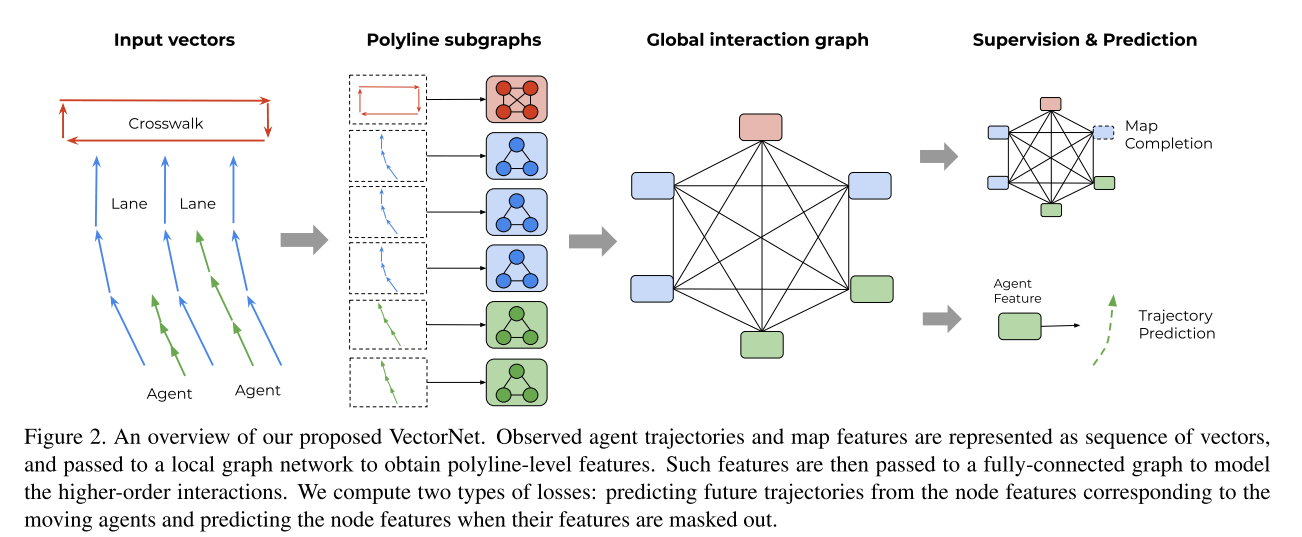image-20201116233730408

### 向量表示

The geographic extent of the road features can be a point, a polygon, or a curve in geographic coordinates. For example, a lane boundary contains multiple control points that build a spline; a crosswalk is a polygon defined by several points; a stop sign is represented by a single point.

lane可以表示为splines，人行道可以表示为一个很多个点组成的polygon，stop sign标记可以表示为单一个点。 对于agent来说，他们的轨迹也是一种splines。 这些元素都可以向量化表示：

for map features, we pick a starting point and di- rection, uniformly sample key points from the splines at the same spatial distance, and sequentially connect the neighboring key points into vectors;

for trajectories, we can just sample key points with a fixed temporal interval (0.1 second), starting from t = 0 , and connect them into vectors.

• 对于地图的特征：选择一个start poine和朝向，等间距均匀采样关键点，并于相邻的关键点相连为向量
• 对于agent轨迹，按照0.1s sample关键点，并将它们连接成向量。

$v_i = [d_i^s, d_i^e, a_i, j]$

• $$d_i^s$$$$d_i^e$$是vector的start和end point的坐标，可以是(x,y)或者(x,y,z)三维的形式
• $$a_i$$则是属性的特征，比如object的类型，轨迹的时间戳，道路的特征，道路限速等
• $$\mathcal{P_j}$$是一个id，表示$$v_i$$属于$$\mathcal{P_j}$$

### Polyline子图构建

$v_i^{(l + 1)} = \phi_{rel} \left( g_{enc}\left(v_i^{(l)}\right), \varphi_{agg}\left(\{v_j^{(l)}\}\right)\right)$

• $$v_i^{(l)}$$代表第i个节点第L层的节点特征
• $$g_{enc}(\cdot)$$代表节点的变换，实践中采用MLP来实现
• $$\varphi_{agg}(\cdot)$$代表聚合，从相邻的节点来获取信息，实践中采用的是max_pooling
• $$\varphi_{rel}(\cdot)$$代表$$v_i$$和周围节点的关系，实践中采用的是concate的操作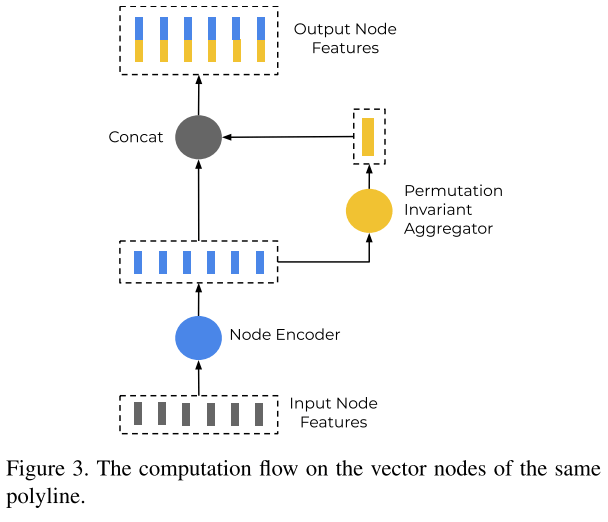image-20201116233919807

### 高阶交互

• $$\{p_i^{(l)}\}$$代表polyline节点的集合

• $$\mathcal{A}$$代表邻接矩阵，实践中采用全链接

• $$GNN(\cdot)$$代表 一层的GNN网络，实践中采用的是self attention layer： $\text{GNN}\left(P\right) = \text{softmax} \left(P_QP_K^T\right) P_V$ 其中，$$P$$是node的feature matrix，$$P_Q,P_k,P_v$$则是它的线性投影。

### 损失函数

$\mathcal{L} = \mathcal{L}_{traj} + \alpha \mathcal{L}_{node}$

• $$\mathcal{L}_{traj}$$ negative Gaussian log-likelihood loss
• $$\mathcal{L}_{node}$$ 是预测的节点和被掩盖节点的huber损失

GaussianLogLikelihood 损失函数为：

$\mathcal{L}(x, y) = -\log p(y) = -\log p\left(y|\mu(x),\Sigma(x)\right)$ where $p(y) = p(y|\mu,\Sigma) = \frac{1}{(2\pi)^{n/2}|\Sigma|^{1/2}} \exp\left\{-\frac{1}{2}(y-\mu)^\top\Sigma^{-1}(y-\mu) \right\}$ Huber比较常见：

$L_{\delta }(y,f(x))={\begin{cases}{\frac {1}{2}}(y-f(x))^{2}&{\textrm {for}}|y-f(x)|\leq \delta ,\\\delta \,|y-f(x)|-{\frac {1}{2}}\delta ^{2}&{\textrm {otherwise.}}\end{cases}}$

## 实验

### Datasets

• Argoverse: 333K 5-second long sequences split into 211K training, 41K validation and 80K testing sequences. (0, 2] seconds are used as observation and (2, 5] seconds for trajectory prediction.
• In-house dataset:2.2M的训练集和0.55M的测试集，轨迹为4s， (0, 1] 作为历史信息 (1, 4] 作为预测值

### Ablation study for the ConvNet baseline

#### Impact of receptive fields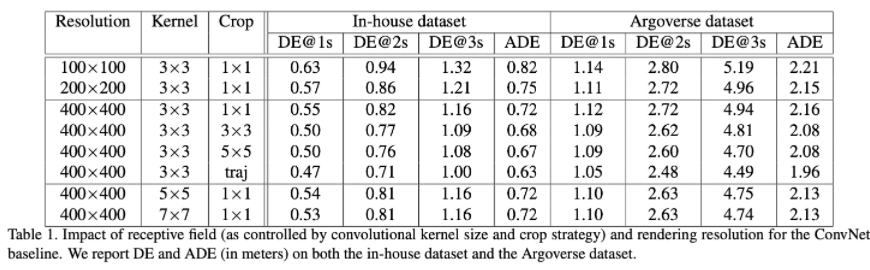image-20201118221744670
• 对比Resolution为400X400的卷积核3，5，7，可以看出更大的卷积核会有更大的性能提升，同时计算量也会增大。
• 从表1的第3行到第6行我们可以看出，较大的裁剪尺寸可以显著地提高性能，同时沿着轨迹裁剪也能得到更好的性能

### Ablation study for VectorNet

#### Impact of input node types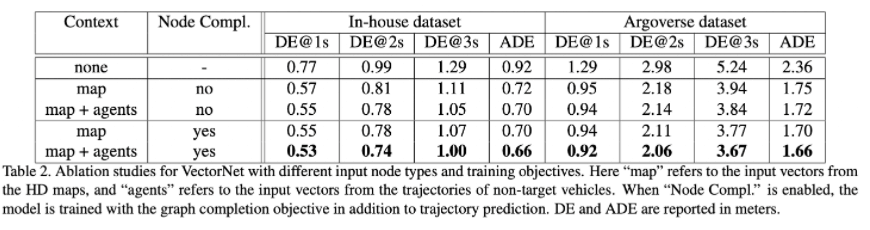image-20201118221833780
• 前三行对应只使用目标车辆的历史轨迹，只添加地图特征以及同时添加轨迹特征。我们可以清楚地看到增加地图特征明显地改善了轨迹预测性能。
• 表2的后四行比较了辅助任务的影响。我们可以看出添加这一任务有助于改善性能，尤其在长期预测。

#### Impact on the graph architectures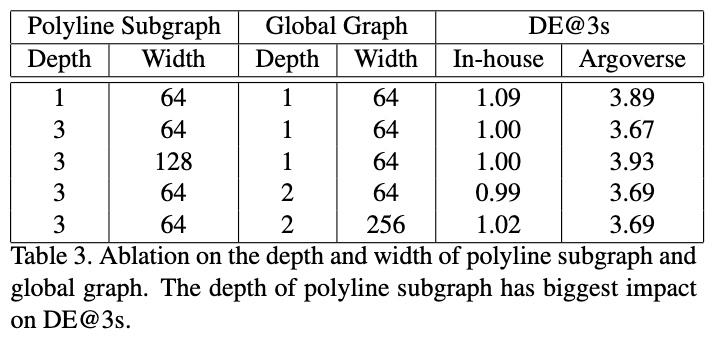image-20201118221853500
• 对于折线子图，三层具有最好的性能，而对于全局图，只需要一层。
• 让MLP变宽并不会带来更好的性能，反而会对Argoverse数据集造成不好的影响

### comparison with ConvNets

##### Comparison of Performance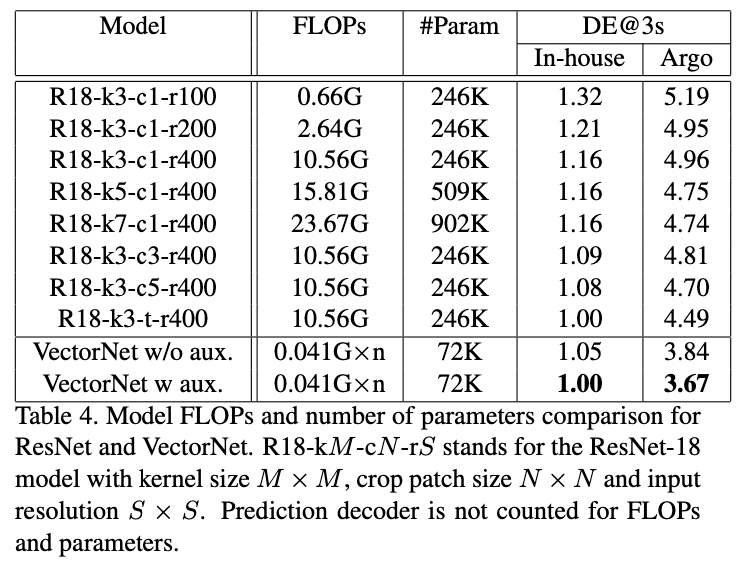image-20201118221923469

#### Comparison of FLOPs and model size

##### Comparison with state-of-the-art methods

VectorNet性能最好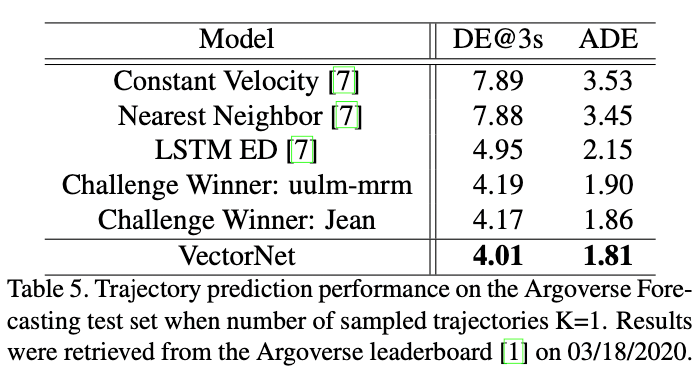image-20201118221955865

## 小结

vectornet是一个比较新颖的特征提取工具，通过将地图与车辆历史轨迹看为"vector"的形式，能较好的学习到环境语义的embedding表达。据说Waymo内部将vector用在高速卡车上。

## 参考文献

1. Gao J, Sun C, Zhao H, et al. VectorNet: Encoding HD Maps and Agent Dynamics from Vectorized Representation[C]//Proceedings of the IEEE/CVF Conference on Computer Vision and Pattern Recognition. 2020: 11525-11533.